Related Articles

# MongoDB Cursor

• Last Updated : 17 Feb, 2021

In MongoDB, when the find() method is used to find the documents present in the given collection, then this method returned a pointer which will points to the documents of the collection, now this pointer is known as cursor. Or in other words we can say that a cursor is a pointer, and using this pointer we can access the document. By default, cursor iterate automatically, but you can iterate a cursor manually which we will discuss later.

Example: In this example, we are working with:

Database: gfg

Collection: student

Documents: Three documents contain the details of the students

Here, we use the following query to display all the documents present in the student collection

`db.student.find().pretty()`

This find() method return a cursor with contain all documents present in the student collection.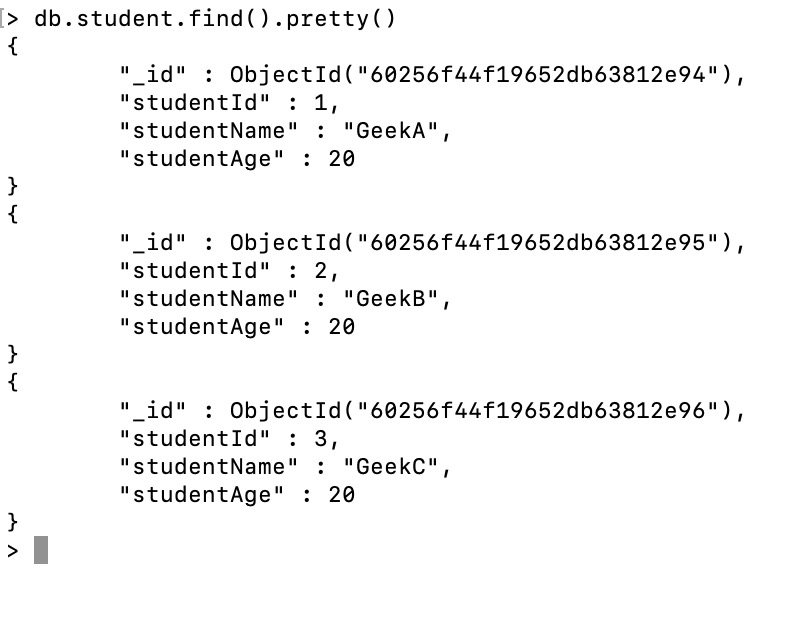### Manually iterating a cursor

In MongoDB, the find() method return the cursor, now to access the document we need to iterate the cursor. In the mongo shell, if the cursor is not assigned to a var keyword then the mongo shell automatically iterates the cursor up to 20 documents. MongoDB also allows you to iterate cursor manually. So, to iterate a cursor manually simply assign the cursor return by the find() method to the var keyword Or JavaScript variable.

Note: If a cursor inactive for 10 min then MongoDB server will automatically close that cursor.

Syntax:

var name = db.collection_name.find()

name

Example :

```var mycursor = db.student.find({studentId:3}).pretty()
mycursor```

Here, we iterate the cursor manually to find the document whose studentId is 3. So, we assigned the cursor returned by the find() method to the JavaScript variable(i.e. mycursor).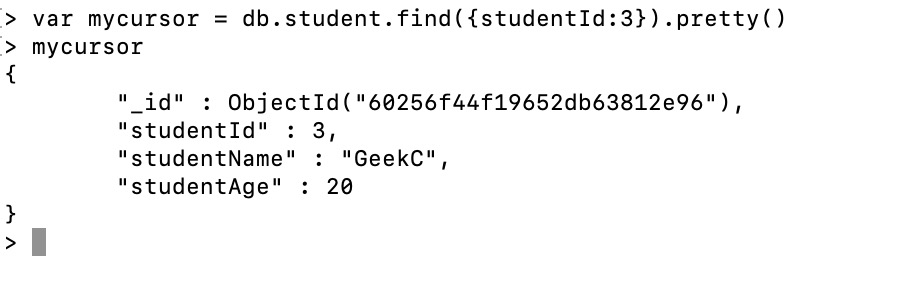Using next() method:

We can also use next() cursor method to access the next document. Let us discuss with the help of an example:

Example:

```var mycursor = db.student.find({studentId:{\$gt:1}});
> while(mycursor.hasNext()){
... print(tojson(mycursor.next()));
... }```

In this example, studentId 2 and 3 documents displays because in the first line we exclusively took the cursor to start with the studentId > 1. So it skipped 1st document and retrieve the remaining documents. Here, print(tojson()) method is used to display the result. You can also use printjson() method to display the result.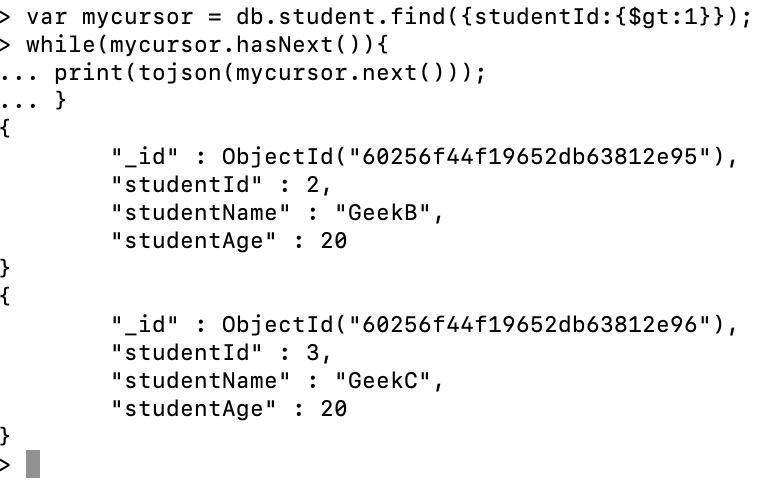Using forEach() method:

We can also use forEach() method to iterate the cursor. This function applies a JavaScript function to each document from the cursor.

Syntax:

db.collection.find().forEach(<function>)

Example:

```var mycursor = db.student.find({studentId:3}).pretty()
mycursor.forEach(printjson)```

Here, first we store the cursor returned by the find() method(i.e., studentId:3) in the mycursor variable. Now, we use forEach() method to iterate the cursor and display the resultant document using printjson.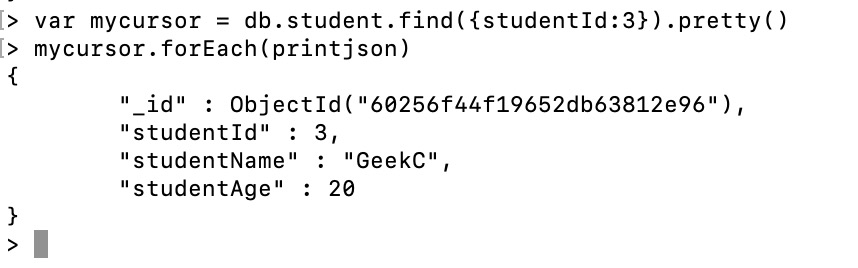Iterator Index :

In mongo shell, you are allowed to iterate the cursor and display the resultant document in the array using toArray() method.

Syntax:

cursor.toArray()

Example:

```var mycursor = db.student.find().pretty()
var docs = mycursor.toArray()
var resultdoc = docs
resultdoc```

Here, first we assign the returned cursor to the var keyword(i.e. mycursor), in the next we create a array from the resultant cursor using toArray() method and assign the result to the var keyword(i.e. docs). Now we access the documents according to their index e.g. var resultdoc = docs, here we display a document whose index is 0.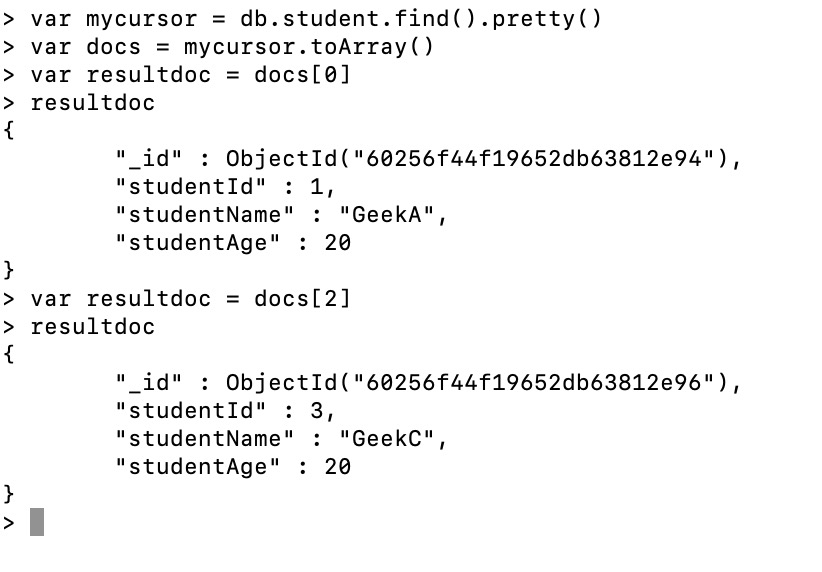Alternate Method:

You can also this method to access a document using index on the cursor.

```var mycursor = db.student.find().pretty()
var resultdoc = mycursor
resultdoc```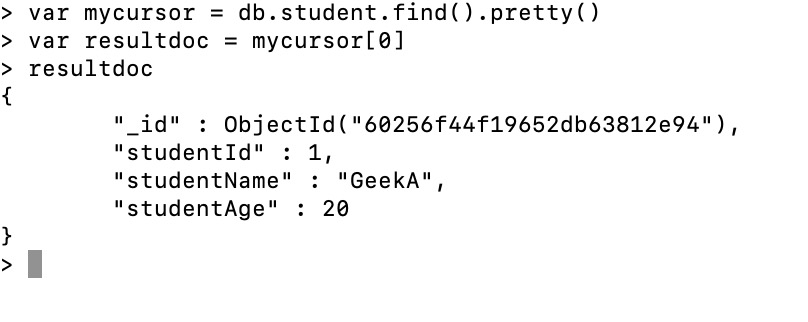### Commonly used Methods

Below are the commonly used cursor methods:

Count cursor:

In order to get the correct documents, we need to know how many documents are present for that collection. To get that we can use the count() method which returns the total number of documents are present in the given collection.

Syntax:

db.collection_name.find().count()

or

db.collection_name.count()

Example:

`db.student.find().count()`

Here, we find the total number of documents present in the student collection using count() method.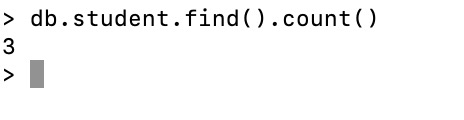Cursor Limit:

The limit() method helps to fetch limited records from a collection. Suppose we have multiple documents, but we want to have topmost or only 2 documents, then by using the limit() method, we can achieve that.

Syntax:

db.collection_name.find().limit(<number>)

Example:

`db.student.find().limit(2).pretty()`

Here, we only display the first two documents from the student collection.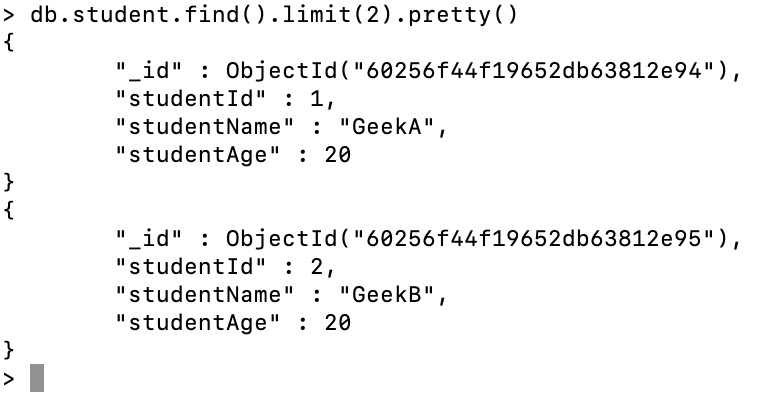Cursor size:

The cursor.size() method will be helpful to return a count of the number of documents which got as the output from db.collection.find() query after applying any cursor.skip() and cursor.limit() methods. Basically, it will filter for the given condition and find the size of the cursor. Hence, it is mentioned as it has applied cursor.skip() and cursor.limit() methods.

Syntax:

db.collection_name.find().size()

Example:

`db.student.find({studentId:1}).size()`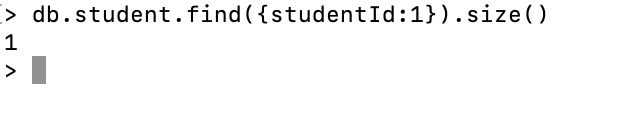Cursor sort:

Usually while verifying documents, if the output is in sorted order, either in ascending or descending order, it will be easier. So we use sort() method to sort the documents. It you want to sort the documents in ascending, then set the value of the field to 1 and in descending, then set -1.

Syntax:

db.collection_name.find().sort(<sort>)

Example:

`db.student.find().sort({studentId:-1}).pretty()`

Here, we sort all the documents present in the student collection in descending order.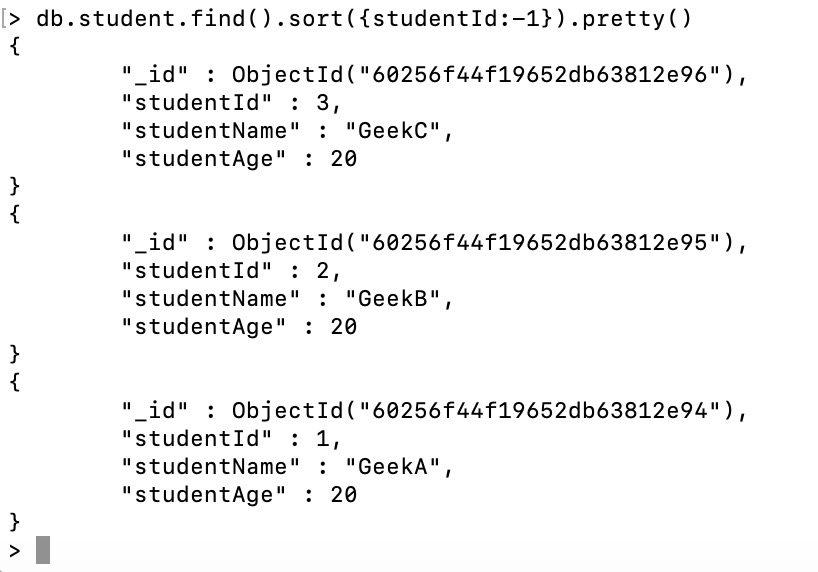Cursor.toArray():

In order to have as an array that contains all documents returned by the cursor, we can use toArray() method.

Syntax:

db.collection_name.find().toArray()

Example:

`db.student.find().toArray()`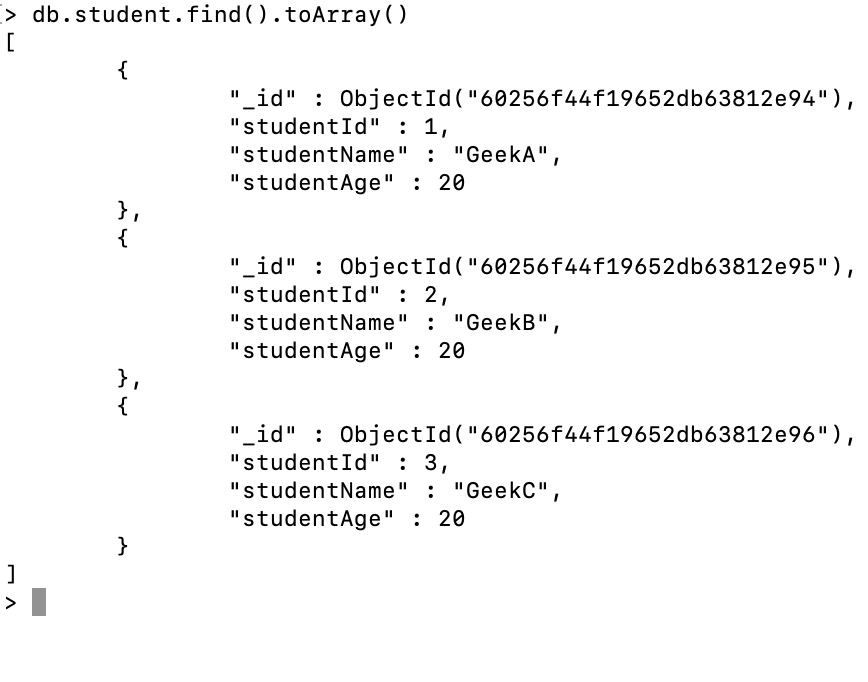Cursor.next:

The next() method is used to return the next document in a cursor. Usually, it will return the first document as that will be the result of the first document in the cursor.

Syntax:

db.student.find().next()

Example:

`db.student.find().next()`

Here, the next() method return the first document from the collection.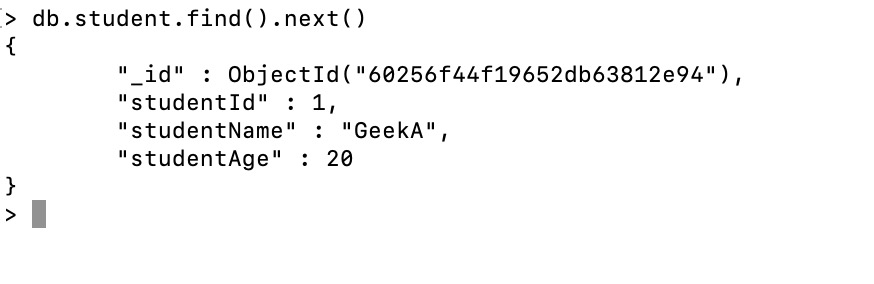### Cursor Method

Cursor Methods modify the way that the underlying query is executed.

My Personal Notes arrow_drop_up